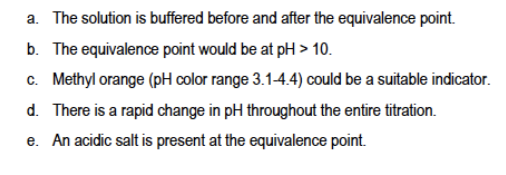Problem: Consider a titration performed using acetic acid and aqueous ammonia. Which of the following statements is correct?

FREE Expert Solution
86% (226 ratings)
Problem Details

Consider a titration performed using acetic acid and aqueous ammonia. Which of the following statements is correct?What scientific concept do you need to know in order to solve this problem?

Our tutors have indicated that to solve this problem you will need to apply the Equivalence Point concept. If you need more Equivalence Point practice, you can also practice Equivalence Point practice problems .

What is the difficulty of this problem?

Our tutors rated the difficulty of Consider a titration performed using acetic acid and aqueous... as medium difficulty.

How long does this problem take to solve?

Our expert Chemistry tutor, Jules took 3 minutes to solve this problem. You can follow their steps in the video explanation above.

What professor is this problem relevant for?

Based on our data, we think this problem is relevant for Professor Morkowchuk's class at UVA.